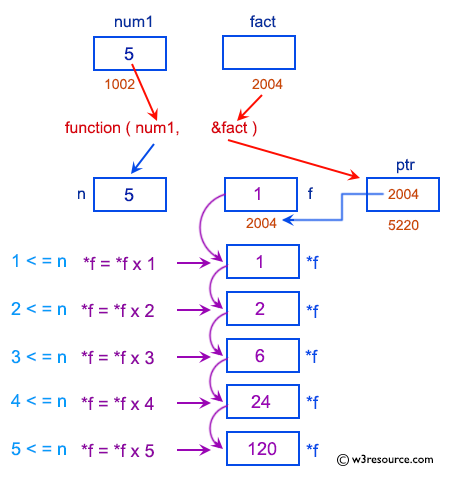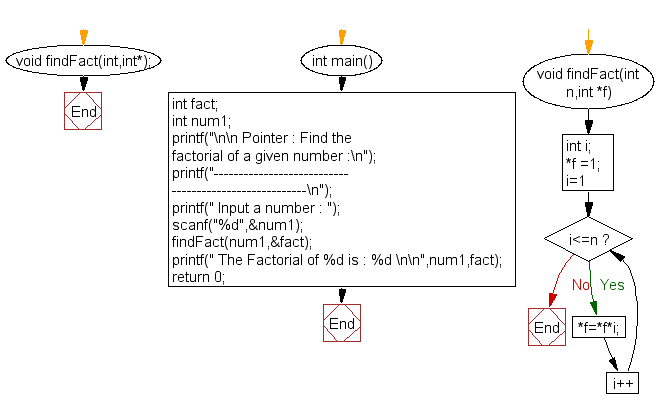﻿ C Program: Find the factorial of a given number - w3resource# C Exercises: Find the factorial of a given number

## C Pointer : Exercise-12 with Solution

Write a program in C to find the factorial of a given number using pointers.

Pictorial Presentation:Sample Solution:

C Code:

``````#include <stdio.h>
void findFact(int,int*);
int main()
{
int fact;
int num1;
printf("\n\n Pointer : Find the factorial of a given number :\n");
printf("------------------------------------------------------\n");
printf(" Input a number : ");
scanf("%d",&num1);

findFact(num1,&fact);
printf(" The Factorial of %d is : %d \n\n",num1,fact);
return 0;
}

void findFact(int n,int *f)
{
int i;

*f =1;
for(i=1;i<=n;i++)
*f=*f*i;
}
```
```

Sample Output:

``` Pointer : Find the factorial of a given number :
------------------------------------------------------
Input a number : 5
The Factorial of 5 is : 120
```

Flowchart:C Programming Code Editor:

Have another way to solve this solution? Contribute your code (and comments) through Disqus.

What is the difficulty level of this exercise?

Test your Programming skills with w3resource's quiz.

﻿

## C Programming: Tips of the Day

How to format strings using printf() to get equal length in the output?

You can specify a width on string fields, e.g.

`printf("%-20s", "initialization..."); `

And then whatever's printed with that field will be blank-padded to the width you indicate.

The - left-justifies your text in that field.

Ref : https://bit.ly/34DMOc3